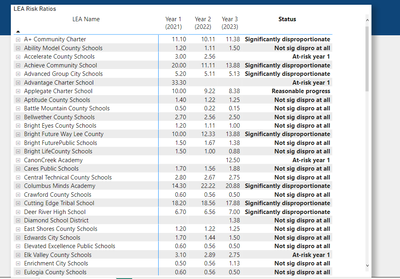cancel
Showing results for
Did you mean:Frequent Visitor

## Create DAX measure using output from another DAX measure to determine an overall status

I have created a DAX measure called 'Risk Ratio' that outputs a risk ratio for each school and school year.  My challenge is to create a new DAX measure using the multi-year Risk Ratio measure to determine an overall status.  I have provided a sample report that has the data and Risk Ratio measure if someone wants to try building the new measure to produce an overall status for each school.  Any help would be greatly appreciated.

1 ACCEPTED SOLUTIONCommunity Support

Hi @Shaker ,

First turn on the column subtotal and change its name to "Status":Then apply the measure to the matrix visual:

``````Measure =
VAR _a =
SUMMARIZE ( 'RDS DimLeas', 'RDS DimLeas'[LEA Name] )
VAR _b =
DISTINCT ( 'Year'[Year] )
VAR _c =
ADDCOLUMNS ( CROSSJOIN ( _a, _b ), "Risk Ratio", [Risk Ratio] )
VAR _d =
SELECTEDVALUE ( 'State Set Threshold'[State Threshold] )
VAR _e =
COUNTROWS ( FILTER ( _c, [Risk Ratio] >= _d ) )
VAR _f =
_c,
"Flag",
IF (
RANKX ( _c, VALUE ( MID ( [Year], FIND ( "(", [Year] ) + 1, 4 ) ),, ASC, DENSE )
= RANKX ( _c, [Risk Ratio],, DESC, DENSE ),
1,
0
)
)
RETURN
SWITCH (
TRUE (),
ISINSCOPE ( 'Year'[Year] ), [Risk Ratio],
NOT ( ISINSCOPE ( 'Year'[Year] ) )
&& _e = 0
&& SUMX ( _c, [Risk Ratio] ) <> BLANK (), "Not sig dispro at all",
NOT ( ISINSCOPE ( 'Year'[Year] ) )
&& _e = 1, "At-risk year 1",
NOT ( ISINSCOPE ( 'Year'[Year] ) )
&& _e = 2, "At-risk year 2",
NOT ( ISINSCOPE ( 'Year'[Year] ) )
&& _e = 3
&& SUMX ( _f, [Flag] ) = 3, "Reasonable progress",
NOT ( ISINSCOPE ( 'Year'[Year] ) )
&& _e = 3
&& SUMX ( _f, [Flag] ) <> 3, "Significantly disproportionate"
)
``````

Final output:Best Regards,

Jianbo Li

If this post helps, then please consider Accept it as the solution to help the other members find it more quickly.

4 REPLIES 4Community Support

Hi @Shaker ,

First turn on the column subtotal and change its name to "Status":Then apply the measure to the matrix visual:

``````Measure =
VAR _a =
SUMMARIZE ( 'RDS DimLeas', 'RDS DimLeas'[LEA Name] )
VAR _b =
DISTINCT ( 'Year'[Year] )
VAR _c =
ADDCOLUMNS ( CROSSJOIN ( _a, _b ), "Risk Ratio", [Risk Ratio] )
VAR _d =
SELECTEDVALUE ( 'State Set Threshold'[State Threshold] )
VAR _e =
COUNTROWS ( FILTER ( _c, [Risk Ratio] >= _d ) )
VAR _f =
_c,
"Flag",
IF (
RANKX ( _c, VALUE ( MID ( [Year], FIND ( "(", [Year] ) + 1, 4 ) ),, ASC, DENSE )
= RANKX ( _c, [Risk Ratio],, DESC, DENSE ),
1,
0
)
)
RETURN
SWITCH (
TRUE (),
ISINSCOPE ( 'Year'[Year] ), [Risk Ratio],
NOT ( ISINSCOPE ( 'Year'[Year] ) )
&& _e = 0
&& SUMX ( _c, [Risk Ratio] ) <> BLANK (), "Not sig dispro at all",
NOT ( ISINSCOPE ( 'Year'[Year] ) )
&& _e = 1, "At-risk year 1",
NOT ( ISINSCOPE ( 'Year'[Year] ) )
&& _e = 2, "At-risk year 2",
NOT ( ISINSCOPE ( 'Year'[Year] ) )
&& _e = 3
&& SUMX ( _f, [Flag] ) = 3, "Reasonable progress",
NOT ( ISINSCOPE ( 'Year'[Year] ) )
&& _e = 3
&& SUMX ( _f, [Flag] ) <> 3, "Significantly disproportionate"
)
``````

Final output:Best Regards,

Jianbo Li

If this post helps, then please consider Accept it as the solution to help the other members find it more quickly.Frequent Visitor

This is incredibly helpful, thank you Jianbo!  Is there a way to make charts to show the Status similar to the one below?  I need to create visuals using the count of schools (LEAs) by Status.

Sample created in Excel...Community Support

Hi @Shaker ,

I'm sorry this question is beyond the original topic of this thread, in order to make the thread more relevant, please consider marking the reply that helped you and creating a new thread for the new question, so that more users can participate and better help others with similar questions.

Best Regards,

Jianbo Li

If this post helps, then please consider Accept it as the solution to help the other members find it more quickly.Frequent Visitor

Understand, I'll send a new request. Thanks for your help!